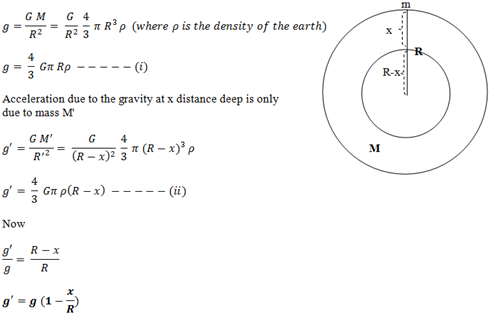# Variation Of ‘g’ Due To Depth

Let us consider the earth to be spherical mass of ‘M’ and radius ‘R’. A body of mass ‘m’ is placed initially on the surface and finally taken x distance deep into the earth.
We know acceleration due to gravity on the surface of earth isThis expression shows that acceleration due to gravity decreases as we go deep into the earth.
At the centre of earth x=R, so g’=0. Hence, the acceleration due to gravity at the centre of earth is zero (0).